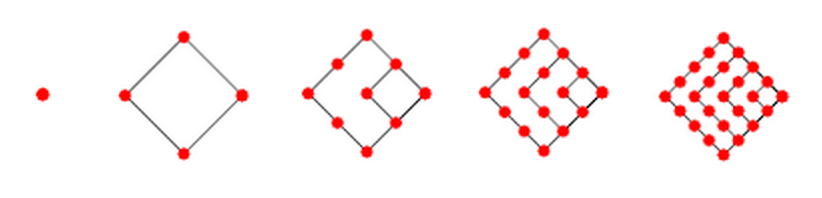#### You may also like### Diophantine N-tuples

Can you explain why a sequence of operations always gives you perfect squares?### DOTS Division

Take any pair of two digit numbers x=ab and y=cd where, without loss of generality, ab > cd . Form two 4 digit numbers r=abcd and s=cdab and calculate: {r^2 - s^2} /{x^2 - y^2}.### Sixational

The nth term of a sequence is given by the formula n^3 + 11n . Find the first four terms of the sequence given by this formula and the first term of the sequence which is bigger than one million. Prove that all terms of the sequence are divisible by 6.

# Square Number Surprises

##### Age 14 to 16Challenge Level

Square Number Surprises printable worksheet

You're probably very familiar with the sequence of Square Numbers: $$1, 4, 9, 16, 25...$$Below are some ideas to explore with square numbers.
For each one, try a few examples and see what you notice.

• Add two consecutive square numbers and then subtract 1
• Square any odd number, then subtract 1
• Multiply two consecutive odd numbers and then add 1
• Multiply two consecutive even numbers and then add 1

Can you explain what you've noticed?
Can you prove that it will always happen?

Can you come up with any Square Number Surprises of your own?

With thanks to Don Steward, whose ideas formed the basis of this problem.